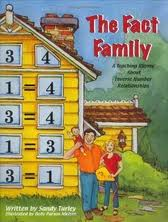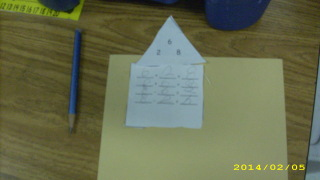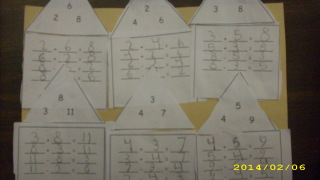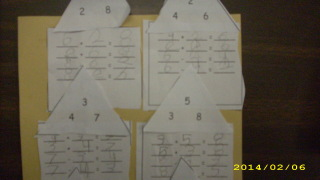# Identify Related Facts - Day 2 of 2

15 teachers like this lesson
Print Lesson

## Objective

The SWBAT identify and create related facts to 20.

#### Big Idea

It's all in the family! In this lesson, students will build fact family "houses" by using 3 numbers and writing the related addition and subtraction sentences.

## Activating Strategy

10 minutes

Start by reading “The Fact Family: A Teaching Rhyme About Inverse Number Relationships” by Sandy Turley.I like to read this story because it easily relates mathematical fact families to real families.

I then give each child 20 connecting cubes – use 2 different colors and give each child 10 of each (ex. 10 red & 10 blue). Write 4 + 5= __ on the board/chart paper.  I then have the students use their connecting cubes to model how to solve the problem.

Next, I write 5 + 4 = __ on the board.  Again, I have the students use their connecting cubes to model how to solve the problem.  I continue this activity by writing the two related subtraction sentences on the board (9-5=__ and 9-4=__).  Ask students if they notice the pattern with the math sentences.  Guide the discussion to the understanding that there is a pattern between the three numbers in a fact family.

In this lesson, children continue to build understanding of the relationship between addition and subtraction.  It is important for first graders to discover a pattern or structure (MP7).  This begins with recognizing that 5+4=9 and 4+5=9 (commutative property).  As children create physical models to solve problems, use questions such as the following to deepen their understanding:

• How do you use cubes to show 4 + 5 = 9? (I start with 4 red cubes and add 5 blue cubes.)

• How do you know your subtraction sentence is a related fact of your addition sentence? (It uses the same numbers.)

• Why do you now have the same number of cubes you started with? (I took away the 5 cubes that I added to the starting number, so I undid the problem.)

## Teaching Strategies

20 minutes

Draw a triangle on the board with the numbers 5, 4, & 9.  Discuss how these numbers are a fact family, and just like our families they are related.  Using the same methods as above, use 9, 1 & 10 and show how they are a related fact family.  (PPT available in the resources section Identify Related facts_houses)

Put students in groups of 2 and have each student select a number between 1 and 10 to add.  Using connecting cubes, have pairs continue model how to add the two numbers.  Guide them to model the subtraction facts in the same family as well.  Use this same strategy and guide pairs through several fact families, walking around to make sure that they understand the concept.

## Independent Practice

30 minutes

Have students complete the attached sheet where they build their own fact family houses.  Students use the three numbers in the “roof” of the house to write the fact family.  Students cut out the “roof” and the house and glue to paper.  Then they write the corresponding fact families on the houses.

Pictures of students working independently:Here are some pictures of student work samples:## Closing/Summarizing

5 minutes

To close out the lesson, I have students get their math journal and identify the two missing related facts in the following fact family:

4 + 9 = 13

13 - 4 = 9Here is some explanation as to why I like to use a close out that involves journaling: